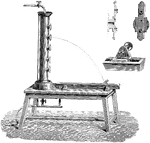### Apparatus for verifying Torricelli's Theorem

This figure represents an apparatus frequently employed for illustrating some of the consequences of…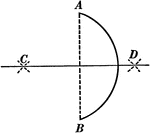### Bisecting an Arc

Illustration used to show how to bisect a given arc.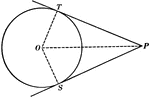### Equal Tangents to Circle Theorem

Illustration used to show that "If two tangents are drawn from any given point to a circle, those tangents…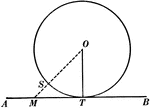### Tangent to Perpendicular Radius Circle Theorem

Illustration used to show that "A tangent to a circle is perpendicular to the radius drawn to the point…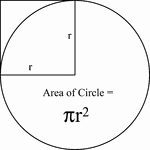### Area Of A Circle

An illustration used to show how the area of a circle is calculated. Area is equal to the product of…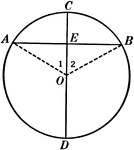### Diameter Perpendicular to a Chord in a Circle

Illustration used to show that "The diameter perpendicular to a chord bisects the chord and also its…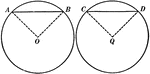### Equal Chords in Equal Circles Theorem

Illustration used to show that "In equal circles, or in the same circle, if two chords are equal, they…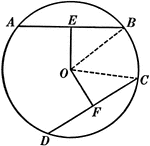### Equal Chords in Equal Circles Theorem

Illustration used to show that "In equal circles, or in the same circle, if two chords are equal, they…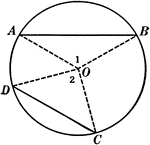### Unequal Chords in Circles Theorem

Illustration used to show that "In equal circles, or in the same circle, if two chords are unequal,…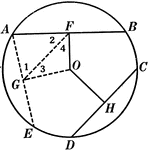### Unequal Chords in Equal Circles Theorem

Illustration used to show that "In equal circles, or in the same circle, if two chords are unequal,…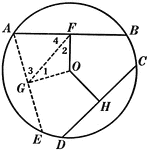### Unequal Chords in Equal Circles Theorem

Illustration used to show that "In equal circles, or in the same circle, if two chords are unequal,…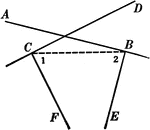### Intersecting Lines Corollary

Illustration used to prove the corollary that "Two lines perpendicular respectively to two intersecting…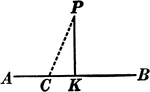### Perpendicular to Line Corollary

Illustration used to prove the corollary that "From a point outside a line there exists only one perpendicular…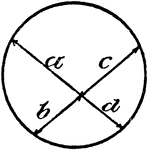### Model Of Geometric Proportions In A Circle

An illustration showing a model of a circle with intersecting chords that illustrates the following…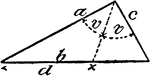### Model Of Geometric Proportions In A Triangle

An illustration showing a model of a triangle that illustrates the following relationship: a:c = d:(b…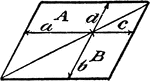### Model Of Geometric Proportions

An illustration showing a quadrilateral model that illustrates the following relationships: a:b = c:d,…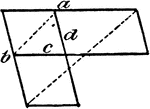### Model Of Geometric Proportions

An illustration showing a model that illustrates the following relationships: a:b = c:d, ad = bc. Product…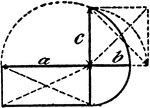### Model Of Geometric Proportions

An illustration showing a model that illustrates the following relationships: a:b = c:b, ab = c²,…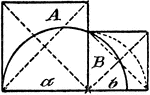### Model Of Geometric Proportions

An illustration showing a model that illustrates the following relationship: A:B = a:b.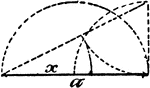### Model Of Geometric Proportions

An illustration showing a model that illustrates the following relationships: a:x = x:a - x, x = √(a²…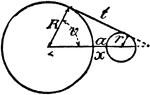### Model Of Geometric Relationships In 2 Circles

An illustration showing a model of 2 circles with tangent lines, diameters, and radii that illustrates…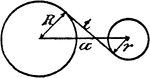### Model Of Geometric Relationships In 2 Circles

An illustration showing a model of 2 circles with tangent lines, diameters, and radii that illustrates…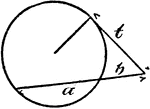### Model Of Geometric Relationships In A Circle

An illustration showing a model of a circle with an exterior angle formed between a tangent and a secant…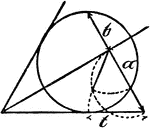### Model Of Geometric Relationships In A Circle

An illustration showing a model of a circle with angles formed between tangents and secants that illustrates…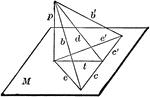### Perpendicular To Line In Plane

"If from the foot of a perpendicular to a plane a straight line is drawn at right angles to any line…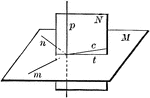### Plane Perpendicular To Line

"All the perpendiculars to a straight line at the same point lie in a plane perpendicular to the line."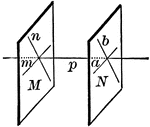### Planes Perpendicular To A Line

"Two planes perpendicular to the same straight line are parallel."### Parallel Lines

"Two straight lines perpendicular to the same plane are parallel."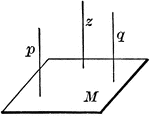### Parallel Lines

"Two straight lines that are parallel to a third are parallel to each other."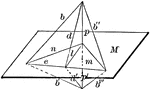### Line Perpendicular To Plane

"If a straight line is perpendicular to each of two straight lines at their point of intersection it…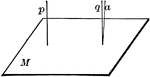### Lines Perpendicular To A Plane

"If one of two parallels is perpendicular to a plane, the other is also."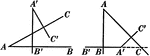### Angles With Perpendicular Sides Are Equal or Supplementary Proof

Illustration used to prove "Two angles whose sides are perpendicular each to each are either equal or…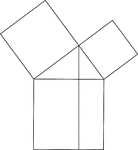### Euclid's Pythagorean Theorem Proof

Illustration used to prove the Pythagorean Theorem, according to Euclid. A perpendicular is drawn from…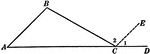### Exterior Angle Proof

Illustration used to prove "An exterior angle of a triangle is equal to the sum of the two remote interior…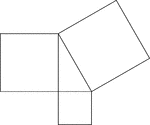### Geometric Pythagorean Theorem Proof

Illustration that can be used to prove the Pythagorean Theorem, the sum of the squares of the legs is…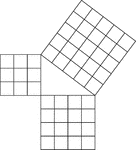### Geometric Pythagorean Theorem Proof

Illustration that can be used to prove the Pythagorean Theorem, the sum of the squares of the legs is…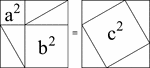### Pythagorean Theorem Proof by Rearrangement

A visual illustration used to prove the Pythagorean Theorem by rearrangement. When the 4 identical triangles…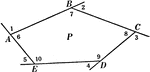### Sum of Exterior Angles of a Polygon Proof

Illustration used to prove "If the sides of any polygon are prolonged in succession one way, no two…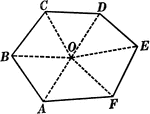### Sum of Interior Angles of a Polygon Proof

Illustration used to prove "The sum of all the angles of any polygon is twice as many right angles as…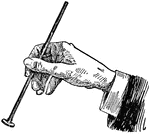### Hand with Proof-plane

"A proof-plane may be made by cementing a bronze cent or a disk of gilt paper to a thin insulating handle,…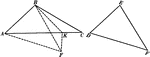### 2 Triangles Theorem

Illustration used to prove that "If two triangles have two sides of one equal respectively to two sides…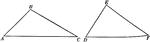### 2 Triangles Theorem

Illustration used to prove that "If two triangles have two sides of one equal respectively to two sides…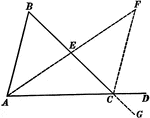### Exterior Angle of Triangle Theorem

Illustration used to prove that "If one side of a triangle is prolonged, the exterior angle formed is…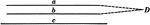### Parallel Lines Theorem

Illustration used to prove that "If two straight lines are parallel to a third straight line, they are…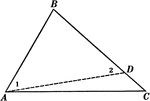### Sides of Triangle Theorem

Illustration used to prove that "If two sides of a triangle are unequal, the angle opposite the greater…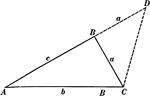### Sides of Triangle Theorem

Illustration used to prove that "The sum of any two sides of a triangle is greater than the third side."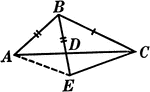### Segments Labeled In A Triangle

Illustration of triangle ABC with BE extended through the triangle at point D. Segment AB is equal to…